# Photovoltaic (PV) - Electrical Calculations

By on

Photovoltaic (PV) cells (sometimes called solar cells) convert solar energy into electrical energy.  Every year more and more PV systems are installed.  With this growing application, it's a good idea for every practicing professional to have an understanding of the calculations associated with PV cells.

There is a vast amount of PV cells in existence, using numerous materials.  At a very simple level, PV cells function by using solar energy to generate electron-hole pairs, which then separate and flow in the external circuit as current. Examining the physics of this of how the current generation works is not the intent of this note, rather we will look at the electrical calculations surrounding the actual application of real systems.

## Electrical Parameters

PV cells are manufactured as modules for use in installations.  Electrically the important parameters for determining the correct installation and performance are:

• Maximum Power - this is the maximum power out put of the PV module (see I-V curve below)
• Open circuit voltage - the output voltage of the PV cell with no load current flowing
• Short circuit current - the current which would flow if the PV sell output was shorted
• Maximum power point voltage - level of voltage on the I-V curve which produces the maximum power
• Maximum power point current - level of current on the I-V curve which produces the maximum power
• Efficiency - measure of the amount of solar energy converted to electrical peak energy

Parameters for PV cells are measured under specified standard test conditions (STC).

STC is generally taken as 1000 W/m2, 25 °C and 1.5 AM (air mass).

The maximum power output is the peak power which a solar cell can deliver at STC.  While common to rate PV installations based on this value, it is unlikely these power levels will be achieved in practice.

For a list of symbols used, see the end of the note.

## Calculation of the output of a system

Example Calculation

120 solar modules, each of 250 Wp and area of 1.67 m2  are connected to form a PV system. The efficiency of the system is 0.75, and the average annual solar radiation is 1487 kWh/m2.  Calculate the expected annual energy production.  Using the above equations: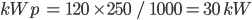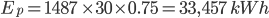If the solar orientation and inclination correction factor is 1.1, what would be the power output: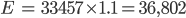Nominal rated maximum (kWp) power out of a solar array of n modules, each with maximum power of Wp at STC  is given by: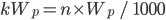- peak nominal power, based on 1 kW/m2 radiation at STC

The available solar radiation (Ema) varies depending on the time of the year and weather conditions.  However, based on the average annual radiation for a location and taking into account the efficiency (η) of the cell, we can estimate an average PV system energy yield:

Note: Ema is given in tables for a particular location and a horizontal plane.

To obtain the anticipated solar radiation requires some research (Internet or local meteorology departments).  If you are using software to perform the calculations, this information is normally provided as part of the program.

The overall efficiency (η) of the solar installation (shading losses, inverter losses, reflection losses, temperature losses, etc.), in a well designed system, these will range from 0.75 to 0.85.

The above calculation is carried out on an annual basis, but could easily be done for any time period (hours, day, month, etc.) by substituting the period mean solar radiation for the annual value.

For maximum power, any solar radiation should strike the PV panel at 90°.  Depending where on the earths surface, the orientation and inclination to achieve this varies.  Software is normally used for the calculation of this or the use of correction coefficients from the concerned location.

## Temperature

As the temperature of PV cells increase, the output drops.  This is taken into account in the overall system efficiency (η), by use of a temperature derating factor ηt and is given by: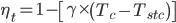Note: power temperature coefficient (ϒ) is typically 0.005 for  crystalline silicon

## Efficiency & Performance

Efficiency: measures the amount of solar energy falling on the PV cell which is converted to electrical energy

Several factors affect the measurement of PV efficiency, including:

• wavelength - PV cells respond differently to differing wave lengths of light, producing varying qualities of electricity
• materials - different PV materials behave differently
• temperature - cells work better at lower temperatures, with efficiency dropping off at higher temperatures
• reflection - any reflected light decreases the efficiency of the cell
• resistance - the cells electrical resistance creates losses, affecting the efficiency

Manufactured PV cells or modules are typically sorted by a binning process into different levels of efficiency.  More efficient cells would have a greater electrical output and hence higher cost.

With the latest development in solar technologies, PV cell are now starting to reach the theoretical maximum limit for semiconductor devices.  The image to the side (click for a larger version) shows the achievable range of efficiencies over differing cell technologies.

In a laboratory, efficiency is measured under standard conditions by the use of I-V curves.  I-V curves are obtained by varying an external resistance from zero (short circuit) to infinity (open circuit).  The illustration shows a typical I-V curve.

Power delivered by the PV cell is the product of voltage (V) and current (I).  At both open and closed circuit conditions the power delivered is zero.  At some point in between (around the knee point) the delivered power is a maximum.

Note: the maximum amount of current that a PV cell can deliver is the short circuit current.  Given the linearity of current in the voltage range from zero to the maximum power voltage, the use of the short circuit current for cable and system dimensioning is reasonable.

### Fill Factor

One way to measure the performance of a solar cell is the fill factor.   This is the ratio of the maximum power to the product of the open circuit voltage and short circuit current: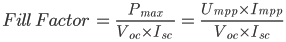The higher the fill factor the better.  As a general rule, commercial PV cells will have a fill factor greater than 0.7.  Cells with factors less than this are not really recommended for practical application in larger electricity generation projects.

### Maximum Power Point Tracking (MPPT)

A PV module's I-V curve can be generated from the equivalent circuit (see next section).   Integral to the generation of tie I-V curve is the current Ipv, generated by each PV cell.

The cell current is dependant on the amount of light energy (irradiance) falling on the PV cell and the cell's temperature.

As the irradiance decreases not only is the amount of power reduce, but the peak power point moves to the left. Similarly as the temperature of the cell increases, the power output lowers and the maximum power point again shifts to the left.

With the maximum power point being a variable quantity, dependant on the solar irradiance and cell temperature, modern inverters have mechanisms to track this and always deliver the maximum possible power from a PV cell.  This is called maximum power point tracking (MPPT).

Note: control systems used to carry out MPPT vary the operation around the current operating point to see if the maximum power point has moved.  They then adjust the operating points accordingly.

## PV Cell Equivalent Circuit

To understand the performance of PV modules and arrays it is useful to consider the equivalent circuit.  The one shown below is commonly employed.

From the equivalent circuit, we have the following basic equations:

The current through the diode is given by Shockley's equation:

Combining the above equations give the PV cell (module) characteristic equation: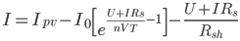Note: the characteristic equations can be used for find both the output voltage and current. Unfortunately, give that voltage and current appear as they do, there is no analytical solution.  Typically numerical methods would be used to solve the equation.

At the limits, it is easy to use the equation to determine the open circuit voltage and short circuit current.  During open circuit conditions, I=0 and the equation reduces to: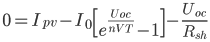Typically Rsh is high compared to the open circuit voltage and the last term can be neglected.  Neglecting the term and rearranging the equation gives:Similarly for the short circuit current, we can set the output voltage to zero, giving: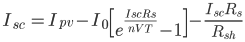The assumption that Rsh is much higher than Rs and that Io is small compared to I.  With these assumptions, the last two terms can be neglected, giving: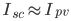The series resistance (Rs), shunt resistance (Rsh) and reverse saturation voltage (Io) are dependent on the area of the PV cell.  Generally the bigger the cell the larger Io (bigger diode junction area) and the lower Rs and Rsh will be.

The characteristic equation can be used to evaluate the effect of various parameters on the performance of the PV cell or module:

• temperature (T) - affects the cell by being part of the exponential term and the value of the reverse saturation voltage.  As the temperature increases, while the exponential will decrease the reverse saturation voltage will increase exponentially. The next effect is to reduce the open circuit voltage of the cell.  Typically the voltage will decrease by 0.35 to 0.5% for each degree increase in temperature.
• series resistance (Rs) - increasing has a similar effect to temperature in that the open circuit voltage will start to drop.  Very high values of Rs will in addition reduced the available short circuit current.
• shunt resistance (Rsh) - decreasing will provide a greater path for the shunt current, again lowering the cell voltage.

## List of Symbols

 General Symbols Impp - current at maximum power, A Isc - short circuit current, A Uoc - open circuit voltage, V Umpp - voltage at maximum power, V PV Systems Ema - mean annual solar radiation, kWh/m2 Ep - estimated peak energy delivered, kWh  kWp - nominal peak energy, kW n - number of modules ηt - temperature derating factor  Pmax - maximum power, W Tc - temperature of the PV cell, K Tstc - temperature of STC, 25 °C, K Wp - peak energy of a single module, W η - efficiency of system ϒ - power temperature coefficient, °C-1 Equivalent Circuit I - current through load, A Id - current through diode, A Ipv - current generated by PV, A Ish - current through the shunt resistor, A Rs - equivalent circuit series resistance, Ω Rsh - equivalent circuit shunt resistance, Ω U - voltage applied to the load, V Ush – shunt voltage, V  Shockley's Diode Equation Io - reverse saturation current, V k - Boltzmann's constant      ==(1.3806488×10−23), J.K-1 n - linearity factor (1 for ideal diode) q - elementary charge       = (1.602176565×10−19), C T – p-n junction absolute temperature, K VT - thermal voltage, V

More interesting Notes:Steven McFadyen

Steven has over twenty five years experience working on some of the largest construction projects. He has a deep technical understanding of electrical engineering and is keen to share this knowledge. About the author

The dc resistance of conductors

This is the first of two posts on the resistance of conductors. In the next post I will look at the ac resistance, including skin effect and we deal with...

Post Authorship

In 2011, with the introduction of it’s Panda search ranking algorithms, Google introduced tools for determining the original author of posts.  The intention...

Cable Insulation Properties

Cable insulation is used to provide electrical separation between conductors of  a cable.  During the historical development of cables, numerous types...

Generation of a Sine Wave

A fundamental concept behind the operation of alternating current systems is that voltage and current waveforms will be sinusoidal – a Sine Wave. This...

Famous Scientists

Here’s list of some famous scientists. Deliberately short, with the aim to provide a quick memory jog or overview. If your looking for more detailed information...

Thomas Edison

American inventor Thomas Alva Edison was born in Milan, Ohio on February 11, 1847. He was the youngest of seven children and received little formal schooling...

Alternating Current Circuits

Alternating current (a.c.) is the backbone of modern electrical power distribution. In this article I’ll be pulling some of the more important concepts...

myElectrical - Cable Sizing Tool Upgrade

Our IEE cable sizing was wrote a few years ago and had become rough around the edges. I thought it was time to give the tool a service. Unfortunately when...

Wiki Depreciation

We have had the Wiki with us for a long time now, but at last I have decided to say bye bye – more details on why below.

Delum, who had watched all in silence, his face empty of expression, now spoke in turn. ' "Lead us, Warleader, into glory."' Reading is something I do...## Have some knowledge to share

If you have some expert knowledge or experience, why not consider sharing this with our community.

By writing an electrical note, you will be educating our users and at the same time promoting your expertise within the engineering community.

To get started and understand our policy, you can read our How to Write an Electrical Note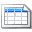Click here to view the notes list in a table formatClick here to view the notes indexed by tag